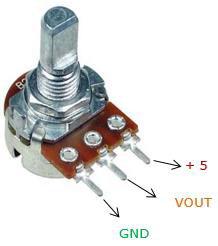# Arduino - 伺服电机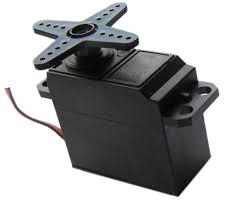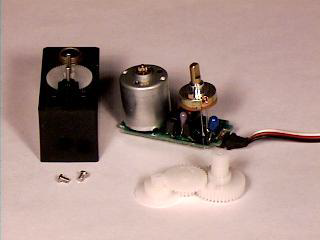## 如何发送伺服转动的角度？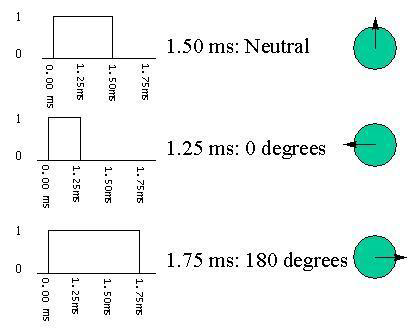## 需要的组件

• 1×Arduino UNO 板
• 1×伺服电机
• 1×ULN2003 驱动 IC
• 1×10KΩ 电阻器

## 程序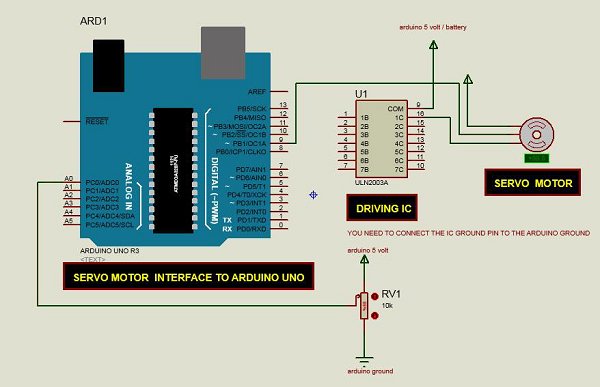## 草图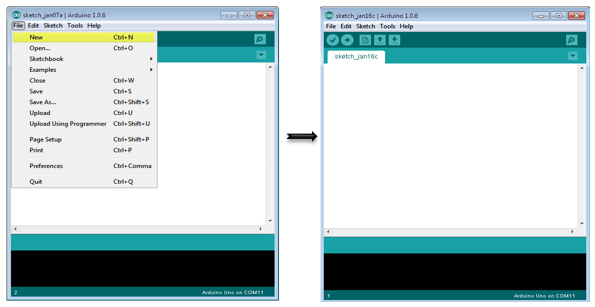## Arduino 代码

/* Controlling a servo position using a potentiometer (variable resistor) */

#include <Servo.h>
Servo myservo; // create servo object to control a servo
int potpin = 0; // analog pin used to connect the potentiometer
int val; // variable to read the value from the analog pin

void setup() {
myservo.attach(9); // attaches the servo on pin 9 to the servo object
}

void loop() {
// reads the value of the potentiometer (value between 0 and 1023)
val = map(val, 0, 1023, 0, 180);
// scale it to use it with the servo (value between 0 and 180)
myservo.write(val); // sets the servo position according to the scaled value
delay(15);
}


## 连接电位器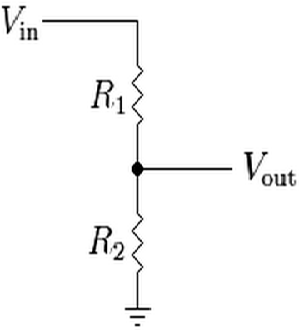$$V_ {out} =（V_ {in} \times R_ {2}）/（R_ {1} + R_ {2}）$$

Vout 是输出电位，取决于串联的输入电压（V in）和电阻（R 1 和 R 2）。这意味着流过 R 1 的电流也将流过 R 2 而不被分开。在上面的等式中，随着 R 2 的值改变，V out 相对于输入电压 V in 相应地缩放。